## 3. 3. 2 Implementation-Based Formulation

The outer integral along the boundary of the simulation domain can be simplified in the following manner by assuming the local support of the basis function: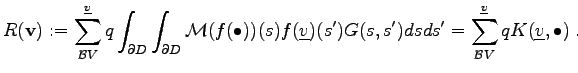(3.49)

The double integral is evaluated over the boundary of the simulation domain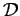.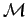denotes the boundary operator,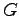denotes the fundamental solution. The basis function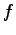is defined by the vertex and stands for the function which equals unity in the argument vertex. The quantity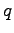defines the weighting coefficient for the respective basis function.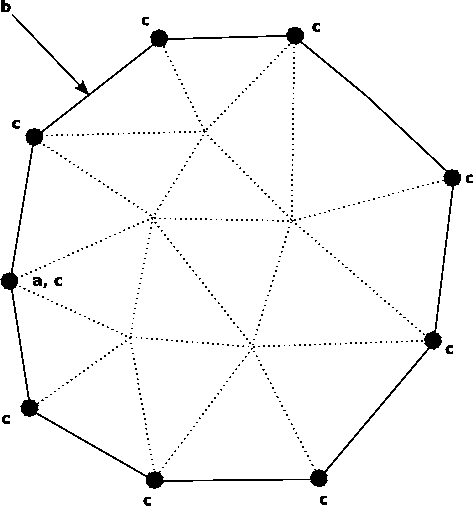The integral expression depends on the type of weighting functions and the shape functions. In this case the Galerkin method is used which means that the shape functions and the weighting functions are equal. Furthermore, the integral expression depends on the choice of the boundary conditions used. Figure 3.9 shows the topological dependences and requirements for the implementation of the boundary element discretization scheme. For the implementation the topological incidence function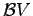is required. Due to the linearity of the boundary condition, the formulation can be re-written in the following form, where the coefficientsand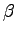can be extracted by treating the expressions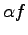and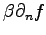of (3.48) separately.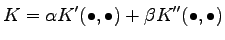(3.50)

It can be seen that the coefficients of the boundary condition are associated with the respective boundary vertices. The evaluation of the integral terms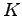depends on the shape of the boundary facets or edges as well as on the shape of the basis functions and the weighting functions.
Michael 2008-01-16